Evaluation Hardware \$199.00••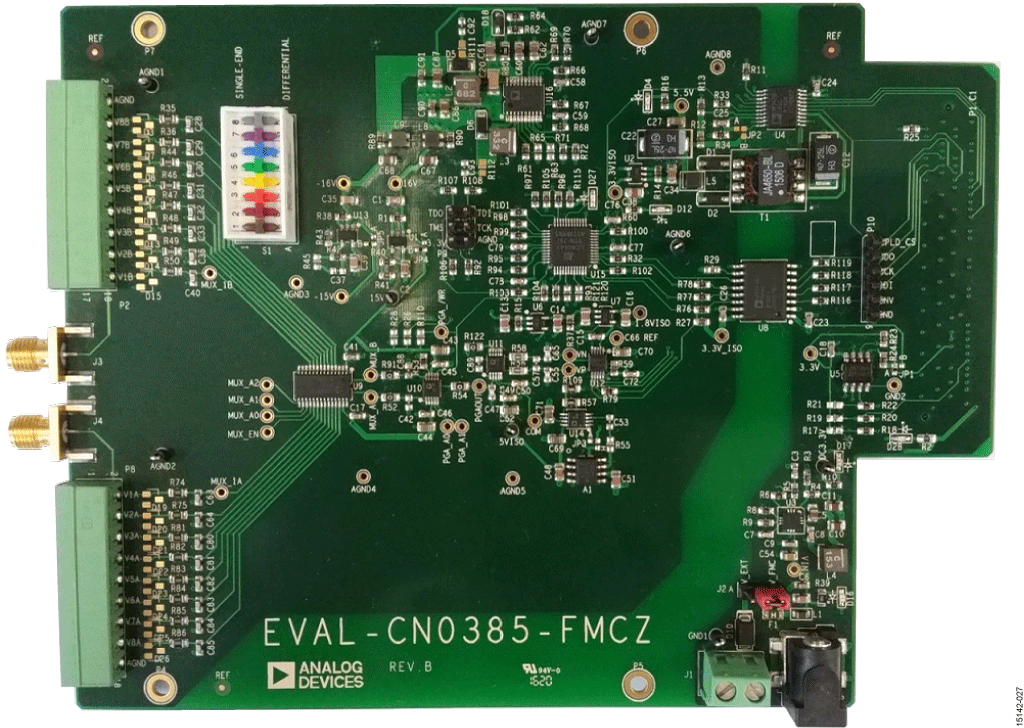# Overview

## Design Resources

### Design & Integration Files

• Schematic
• Bill of Materials
• Gerber Files
• Layout Files (Allegro)

### Evaluation Hardware

Part Numbers with "Z" indicate RoHS Compliance. Boards checked are needed to evaluate this circuit

• EVAL-CN0385-FMCZ (\$199.00) Isolated, Multichannel Data Acquisition System with PGIA
• EVAL-SDP-CH1Z (\$199.00) Eval Control Board

## Features & Benefits

• Multichannel Isolated Data Acquisition System
• Industrial Signal Levels
• 18-bit, 2MSPS Conversion
• 750kHz Channel Switching Rate

# Circuit Function & Benefits

The circuit shown in Figure 1 is a cost effective, isolated, multi- channel data acquisition system that is compatible with standard industrial signal levels. The components are specifically selected to optimize settling time between samples, providing 18-bit performance at channel switching rates up to approximately 750 kHz.

The circuit can process eight gain-independent channels and is compatible with both single-ended and differential input signals.

The analog front end includes a multiplexer, programmable gain instrumentation amplifier (PGIA); precision analog-to- digital converter (ADC) driver for performing the single-ended to differential conversion; and an 18-bit, 2.0 MSPS precision PulSAR® ADC for sampling the signal on the active channel. Gain configurations of 0.4, 0.8, 1.6, and 3.2 are available.

The maximum sample rate of the system is 2 MSPS in turbo mode, and 1.5 MSPS in normal mode. The channel switching logic is synchronous to the ADC conversions, and the maximum channel switching rate is 1.5 MHz. A single channel can be sampled at up to 2 MSPS with 18-bit resolution in turbo mode. Channel switching rates up to 750 kHz also provide 18-bit performance.Figure 1. Isolated Multichannel Data Acquisition Simplified Circuit (All Connections and Decoupling Not Shown)

# Circuit Description

The circuit shown in Figure 1 is an isolated multichannel data acquisition signal chain consisting of a multiplexer, programmable gain stage, ADC driver, and a fully differential, precision, successive approximation register (SAR) ADC. The channel switching and gain switching is synchronized to the conversion period of the ADC.

The system can monitor up to eight channels using a single ADC, reducing component count and cost compared to systems with one ADC per channel. Each channel can be configured with a different gain, allowing for flexibility of input ranges. It is manipulated by the complex programmable logic device (CPLD) which can be configured in the Labview graphical user interface (GUI). The effective sample rate for each channel is equal to the sample rate of the ADC divided by the total number of channels being sampled.

The maximum sample rate of the system is limited by the settling time of the components (such as the programmable gain amplifier (PGA) bandwidth and RC filter bandwidth) in the analog front end and the isolated digital interface clock rate which runs at 75 MHz. Multiplexed signals are discontinuous in nature, resulting in potentially large voltage steps between sampling intervals. The components in the signal chain must be given adequate time to settle to these steps before the ADC performs a conversion. To maximize the time given for the signal to settle, the multiplexer channels are switched immediately after the ADC begins a new conversion.

The board power supply can take a dc input from 5 V to 12 V at the dc jack or 12 V from the SDP-H1 controller board. The ADP2441 dc-to-dc converter generates 3.3 V for the digital interface and the ADuM3470 primary supply input. The ADP5070, ADP7118, and ADP7182 are used to generate positive and negative ±15 V supplies. The ADP7118 is used to generate 5 V, 3.3 V, and 1.8 V for the analog and digital power supplies. The ADuM141E is selected for isolated high speed SPI communication. It has 150 Mbps maximum data rate, low propagation delay, and low dynamic power consumption.

#### Component Selection

The ADG5207 is a high voltage, latch-up proof, 8-channel differential multiplexer. The ultralow capacitance and charge injection of these switches make them ideal solutions for data acquisition and sample-and-hold applications, where low glitch and fast settling are required. A switching network at the inputs of the ADG5207 adds compatibility with both single-ended and differential input signals. The active channel is selected via the address pins of the device, which are controlled by the CPLD, and which can be configured in the GUI.

The AD8251 is a programmable gain instrumentation amplifier that provides selectable gain settings of 1, 2, 4, and 8. The higher gain settings boost smaller input signals to the full-scale input range of the AD4003. Each gain setting has its own suitable input range, which is shown in Table 1.

 Gain Full-Scale Input Range 0.4 ±10.24 V 0.8 ±5.12 V 1.6 ±2.56 V 3.2 ±1.28 V

The AD8475 funnel amplifier provides high precision attenuation (0.4×), accurate common-mode level shifting, and single-ended to differential conversion. Its low output noise spectral density (10 nV/√Hz) and fast settling time (50 ns to 0.001% for a 2 V output step) make it well suited to drive the AD4003.

The AD4003 is a fully-differential, 2 MSPS, 18-bit precision SAR ADC that features a typical signal-to-noise ratio (SNR) of 98 dB when using a 4.096 V reference. The AD4003 is also low power, and only consumes approximately 17 mW at full throughput. Its power consumption scales with throughput, and can operate at lower sample rates to cut its power use (for example, 0.17 mW at 100 kSPS).

#### System DC Accuracy Errors

Figure 2 shows the ideal transfer function of the data acquisition system.

Each of the components in the data acquisition signal chain adds its own offset error and gain error that cause the real transfer function of the system to deviate from the ideal transfer function shown in Figure 2. The cumulative effects of these errors can be measured at a system level by comparing known dc inputs near zero and full scale at the input to the ADG5207 (RC filter if it is present) and the resulting output codes from the AD4003 to obtain a system calibration factor.

Offset Error Measuremennt
For ideal bipolar, differential ADCs, a 0 V differential input results in an output code of 0. Real ADCs typically exhibit some offset error (εb), which is defined as the deviation between the ideal output code and the measured output code for a 0 V input.

The offset error for the data acquisition system can be found by grounding its input and observing the resulting output code. This error varies between each of the gain settings of the AD8251 and between each of the channels of the ADG5207. Offset error is therefore measured for each of the channels in all four gain configurations.

Because the system monitors multiple channels, it is also important to quantify the amount by which the offset error deviates between channels. Offset error match (Δεb, MAX) is a measure of the max-imum deviation between the offset error of each of the channels and the average offset error of all of the channels. Offset error match is calculated using the following equation:where εb,i and εb,j are the offset errors for the i and j channels, respectively.

This offset error match can be found for each of the gain configurations. Note that offset error can be expressed either in codes or volts.

Gain Error Measurement
Error in the gain of the system also contributes to overall system inaccuracy. The ideal transfer function of the AD4003 is shown in Figure 2, where the −217 and 217 − 1 output codes correspond to a negative full-scale input voltage (−FS) and a positive full-scale input voltage (+FS), respectively; however, the combination of offset error (εb) and gain error (εm) results in a deviation from this relationship.

Gain error can be expressed as a percentage error between the actual system gain and the ideal system gain. The more common expression is in percent full-scale error (%FS), which is a measure of the error between the ideal and actual input voltages that produces the 217 − 1 code.

The ideal full-scale input voltage (VFS, IDEAL) is a function of the resolution of the ADC (18-bits for the AD4003) and the accuracy of the reference voltage (VREF). Errors in the voltage reference translate to gain errors in the ADC. To decouple reference errors from ADC gain error, VREF is measured using a precision multimeter. The ideal full-scale input voltage can then be calculated using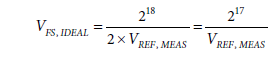The actual system gain can be found by calculating the slope of the linear regression of a group of several input voltages (mLR) and the resulting output codes:The real full-scale input voltage (VFS, REAL) can then be calculated usingThe gain error (expressed in %FS error) can then be calculated usingThe gain error of the system varies with the gain of the AD8251, but is channel independent. Therefore, gain error is measured for each of the four gain configurations, but only using one of the ADG5207 channels in this system.

System Noise Analysis
One of the key design goals in precision data acquisition systems is achieving a high SNR, which can be achieved by increasing the full-scale signal amplitude and/or by decreasing the noise power generated by the components in the system.

The total noise power present in the system can be found by taking the root sum square (rss) of the noise power contributed by its individual components, referred to the input of the AD4003:The expected SNR of the system (SNREXPECTED) can then be found using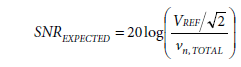The expected noise contributions for each component in the system and the resulting expected SNR performance of the whole system is shown in Table 2. The total system noise calculation ignores thermal noise contributed by the passive components in the system.

The noise of the AD4003 ADC is a function of both its inherent quantization error and noise caused by internal components (such as passive components producing thermal noise). The rms input voltage noise of the AD4003 can be calculated from its specified SNR usingThe SNR for the AD4003 (SNRAD4003) is specified as approximately 98 dB for a 4.096 V reference.

The single-pole RC filter at the input of the AD4003 limits the wideband noise from the upstream components. A smaller filter bandwidth improves SNR by further limiting noise power; however, its time constant must also be sufficiently short to settle voltage kickbacks due to charge injections that occur as the AD4003 inputs reconnect to the front-end circuitry during the acquisition phase. The appropriate bandwidth for the system is at least 5 MHz (for more information, see the Analog Dialogue article, Front-End Amplifier and RC Filter Design for a Precision SAR Analog-to-Digital Converter).

Noise Due to the AD8475 Funnel Amplifier
The rms noise contributed by the AD8475 (vn, AD8475) is a function of its referred to output noise spectral density (NSD) (eAD8475) and the RC filter bandwidth at the input to the AD4003 (BWRC):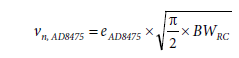Noise Due to the AD8251 Instrumentation Amplifier
The AD8251 functions as a gain stage that improves SNR for small amplitude signals by boosting their amplitude to more closely fill the ±VREF range at the input to the AD4003. Ideally, if the system gain increases by a factor of G, the SNR (in dB) of the input signal improves byThis level of improvement is not achievable in reality, however, because wideband noise is also amplified by the noise gain of the circuit. Fortunately, this degradation is not as large as the improvement due to signal gain.

The rms noise contributed by the AD8251 is a function of its referred to input NSD (eAD8251), its gain setting (GAD8251), the attenuation factor of the AD8475 (GAD8475), and the noise filter bandwidth at the input of the AD4003: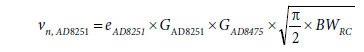The value of eAD8251 is also dependent on the AD8251 gain; the value of eAD8251 can be found in the AD8251 data sheet.

Noise Due to the ADG5207 Multiplexer
The NSD and resulting rms noise contributed by the ADG5207 can be found by using the Johnson/Nyquist noise equation, because the device acts like a series resistance between the source and the rest of the analog front end:and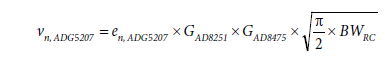The resistance of each channel (RON) can be found in the ADG5207 data sheet.

A summary of the calculated noise performance of the system is shown in Table 2. The largest contributors to the total noise are the AD8251 in-amp and the AD4003 ADC.

#### Settling Time Analysis

When the circuit shown in Figure 1 is sampling multiple channels, each of the different inputs are merged into a time-division multiplexed signal by the ADG5207. Multiplexed signals are discontinuous in nature, and typically have large voltage steps occurring in short time intervals. For the system in Figure 1, the voltage differential between two consecutive channels may be as large as 20 V at the inputs of the ADG5207, and the time allotted for settling is only as long as the sampling period.

Figure 3 shows the settling time model of the circuit in Figure 1. Each of the components in the system has its own settling characteristics (see the following sections).

Settling time is defined as the time required for the analog front-end circuitry to settle an input step to a certain precision. This precision is usually specified in percent error (for example, 0.1% or 0.01%); however, in conversion systems, it is also helpful to relate it to resolution. For example, settling to a 16-bit resolution is roughly equivalent to settling to 0.001%. Table 3 shows the relationship between settling to percent error and to resolution for a single-pole system.

 Resolution, No. of Bits LB (%FS) No. of Time Constants = –ln (%Error/100) 6 1.563 4.16 8 0.391 5.55 10 0.0977 6.93 12 0.0244 8.32 14 0.0061 9.70 16 0.00153 11.09 18 0.00038 12.48 20 0.000095 13.86 22 0.000024 15.25

Estimating the settling time of an analog front end with multiple components is not trivial for a variety of reasons. First, many devices do not specify settling characteristics to very high precision. Settling time for an active device is also not linearly related to settling precision, and it may take up to 30 times as long to settle to 0.01% as to 0.1%. The settling time can be due to long-term thermal effects inside the amplifier. Settling time is also dependent on the load that the device is driving, and settling time is generally not characterized for multiple load conditions.

Measuring high precision settling is also difficult without a specialized characterization platform, because of the effects of oscilloscope overdrive and sensitivity, and the difficulty of generating an input pulse with sufficient rise time and settling time.

Settling time can be estimated provided certain bounds and assumptions are used in analyzing the circuit. The total settling time can be calculated by taking the rss of the settling times of the individual components:The maximum throughput of the system is inversely proportional to the total settling time:The equivalent circuit for a CMOS switch can be approximated as an ideal switch in series with a resistor (RON) and in parallel with two capacitors (CS, CD). The multiplexer stage and associated filters can therefore be modeled as shown in Figure 4.

Each channel functions similarly to an RC circuit having an associated time constant that dominates settling time. Dynamically switching channels complicates signal settling; at the time channels are switched, the difference between the previous output and the current input produces a kickback transient. This kickback is similar to the one that occurs at the input to the AD4003 as it enters the acquisition phase. For a more detailed description, see the Analog Dialogue article, Front-End Amplifier and RC Filter Design for a Precision SAR Analog-to-Digital Converter.

The circuit in Figure 4 was simulated using NI Multisim™, as shown in Figure 5, with the following component values from the respective device data sheets:

• RON = 250 Ω
• CS = 3.5 pF
• CD = 36 pF
• RIN||CIN = 1.25 GΩ||2 pF

The input resistance of the AD8251 (RIN) is sufficiently large (1.25 GΩ) to be omitted from simulation.

The simulation results are shown in Figure 6. The time required for the output of the ADG5207 to settle to 0.001% of 10 V is tS_ADG5207 = 188 ns.

The AD8251 data sheet specifies its settling time for a variety of input voltage step sizes down to a 0.001% error for each gain configuration. Given a load of 10 kΩ and gain setting of 1, the AD8251 can settle a 20 V step at its output to 0.001% in approximately 1 μs. The gain of 1 setting requires the most settling time; therefore, the settling time analysis uses 1 μs.

However, the 1 μs number may not be accurate when the AD8251 is driving one of the inputs of the AD8475, which has an input impedance of 2.92 kΩ instead of 10 kΩ. It is also not possible to ascertain settling time of the AD8251 to 18-bit resolution, because of the nonlinear relationship between settling time and precision. Therefore, the best settling time estimation is 0.001% error (or 16-bit resolution).

The AD8475 has a settling time specification of 50 ns to 0.001% for a 2 V differential output step. The maximum voltage step size expected on the outputs of the AD8475 is twice the reference voltage (VREF), or approximately 8 V. Assuming that the settling time is proportional to the output voltage step, the settling time to 0.001% (16 bits) for an 8 V step is approximately 200 ns (4 × 50 ns).

The settling time of each amplifier is, therefore,

Settling Time of the RC Noise Filter and AD4003
Figure 7 shows the equivalent circuit of the inputs of the AD4003. REXT and CEXT are the components in the RC wideband noise filter in front of the ADC. RIN and CIN are the input resistance and capacitance of the AD4003, respectively. CIN is mainly the internal capacitive digital-to-analog converter (DAC). CPIN is primarily the pin capacitance, and is ignored. The values for these components are as follows:

• REXT = 200 Ω
• CEXT = 120 pF
• RIN = 400 Ω
• CIN = 40 pF

The AD4003 employs an internal capacitive DAC and a charge redistribution algorithm to determine its output code. The conversion process contains two phases, acquisition and conversion. During acquisition, the capacitive DAC is connected to the input terminals of the AD4003. During conversion, it is disconnected from the input terminals, and internal logic performs the charge-redistribution algorithm. Compared to other PulSAR ADCs, the AD4003 has a much shorter conversion time, and it allows the user to return to acquisition phase before the end of conversion. Therefore, if the user runs the ADC at slower throughput, there is have more time to settle the kickback.

The signal must be settled by the end of the acquisition phase for an accurate conversion. To maximize the time given for the signal to settle, the multiplexer switches channels immediately after the AD4003 begins its conversion phase.

In addition to settling from the multiplexed signal from the output of the AD8475, the RC noise filter and AD4003 inputs also need to settle to the voltage kickback that occurs at the beginning of the acquisition phase. For more information, see the Analog Dialogue article, Front-End Amplifier and RC Filter Design for a Precision SAR Analog-to-Digital Converter.

The settling time for the circuit in Figure 7 was simulated in NI Multisim, as shown in Figure 8. V1 represents the maximum voltage step expected at either input of the AD4003 (from a single-ended output of the AD8475). CNV and S1 simulate the AD4003 switching from the conversion phase (occurring when V1 changes value) to the acquisition phase (300 ns after start of conversion). CNV keeps S1 open until 300 ns after V1 steps from 0 V to 4 V to represent the transition from the conversion phase to the acquisition phase. ADC_IN is the voltage that is sampled by the AD4003 on a CNV rising edge.

The settling time for this portion of the system is equal to the time between V1 switching to 4 V (at TIME = 0, see Figure 9) to ADC_IN settling to 0.001% of 4 V.

The simulation results are shown in Figure 9. The time taken for the output to settle to 0.001% of 4 V is tS_AD4003= 711 ns.

Total System Settling Time
The total settling time of the entire circuit shown in Figure 1 can now be estimated by calculating the rss of the settling times for each component: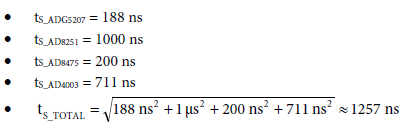The expected maximum channel switching sample rate of the system is thenOffset and Gain Error Results
Table 4 shows the offset error measured (in LSBs) for each of the channels in each gain configuration for the circuit in Figure 1. Table 4 also shows the average offset error of all of the channels for each gain configuration.

The offset errors were measured by grounding all of the channel inputs and collecting and averaging 32,768 samples taken on each of the channels in each gain configuration.

 Gain Channel 1 Channel 2 Channel 3 Channel 4 Channel 5 Channel 6 Channel 7 Channel 8 Channel Average 0.4 1.34 1.33 1.31 1.36 1.44 1.45 1.46 1.48 1.40 0.8 1.98 1.99 2.02 2.06 2.00 1.98 1.99 1.97 2.00 1.6 3.25 3.19 3.22 3.19 3.17 3.08 3.13 3.14 3.17 3.2 5.57 5.66 5.67 5.55 5.57 5.50 5.54 5.52 5.57

Table 5 shows the gain error measured for each of the gain configurations for the circuit in Figure 1. The %FS error was found using the analysis methods described previously, and the actual gain in V/V was calculated by subtracting this error from the ideal gain.

 Gain Gain Error (%FS) 0.4 0.02 0.8 0.02 1.6 0.03 3.2 0.02

#### Performance Results Without Channel Switching

Figure 10, Figure 11, Figure 12, and Figure 13 show the fast Fourier transform (FFT) plots for a 10 kHz, full-scale, sine wave input on a single channel for gain configurations of 0.4, 0.8, 1.6, and 3.2, respectively. Table 6 shows the SNR and rms noise measured for each of the gain configurations.

 Gain SNR (dB) RMS Noise (μV rms) THD (dB) 0.4 93.9 55.2 –99.2 0.8 92.8 62.6 –98.2 1.6 90.6 80.7 –97.0 3.2 88.0 108.9 – 94.6

The input signal was supplied by an Audio Precision SYS-2700 series signal generator, with the board set in differential input mode. Figure 14 shows total harmonic distortion (THD) measurements vs. the frequency of the input signal for each gain configuration.Figure 10. FFT for 10 kHz, 20 V p-p Input for Gain = 0.4 on Single, Static Channel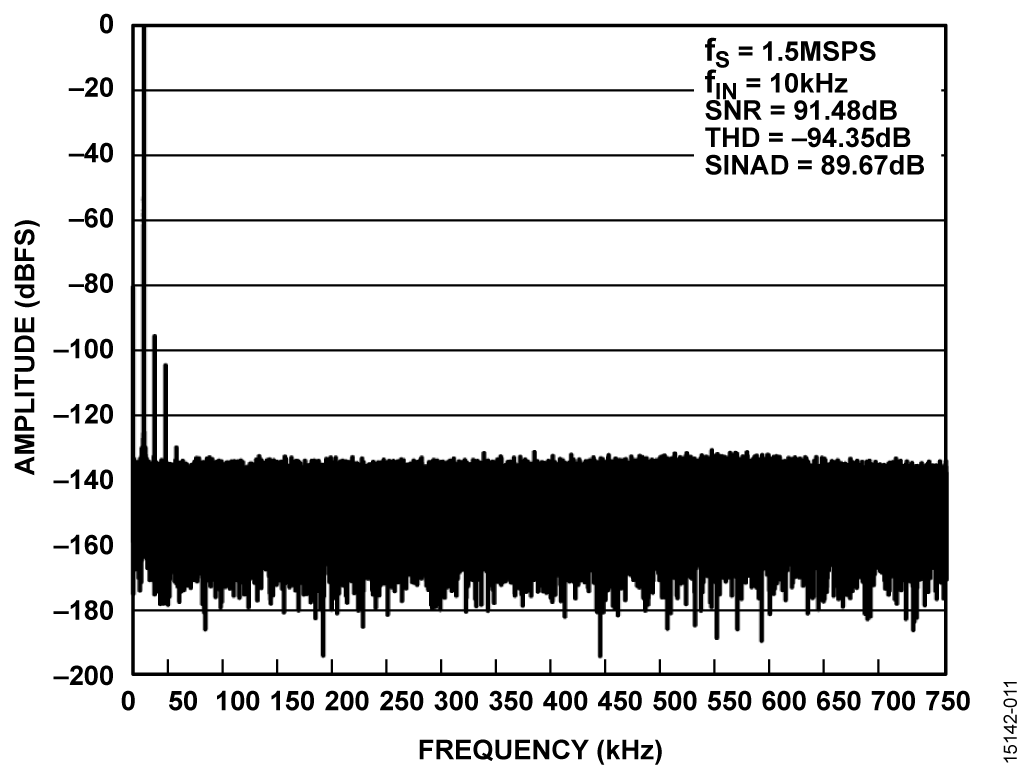Figure 11. FFT for 10 kHz, 10 V p-p Input for Gain = 0.8 on Single, Static ChannelFigure 12. FFT for 10 kHz, 5 V p-p Input for Gain = 1.6 on Single, Static ChannelFigure 13. FFT for 10 kHz, 2.5 V p-p Input for Gain = 3.2 on Single, Static ChannelFigure 14. THD Measured for Various Input Frequencies on a Single, Static Channel

#### System Performance with Channel Switching

Several tests were performed to evaluate the performance of the system when scanning multiple channels. Experiments using precision dc sources measured the error in output code with respect to sample rate (see the Circuit Note CN-0269 for similar tests) and voltage step size between channels. AC performance was also measured for switching between two out of phase, full-scale inputs from a precision ac source (Audio Precision AP SYS-2712).

Figure 15 and Figure 16 show the test setup for dc and ac performance tests, respectively. The channel switching rate is the rate at which the ADG5207 switches from one channel to another, and is equivalent to the sample rate of the AD4003.

The dc tests involved varying the voltage step size between the two channels and the channel switching rate. The channel switching rate was varied from 50 kHz to 1 MHz in 50 kHz increments. The voltage step size was varied over different ranges for each of the gain configurations. A mean code result was measured for each channel for each voltage step size and channel switching rate by averaging 8,192 samples taken on each channel. A mean code result was also measured for each channel in the static case (no switching between channels). The mean code errors discussed below were found by taking the difference between the mean codes measured for the static case and for the switching channels.

Figure 17, Figure 18, Figure 19, and Figure 20 show the mean code error for various voltage step sizes at several switching rates in each of the four gain configurations. Figure 21, Figure 22, Figure 23, and Figure 24 show the mean code error for full-scale voltage steps at various switching rates in each of the four gain configurations.

The mean code error increases as the voltage step size and channel switching rate increase. This increase is caused by the combined slew and settling time limitations of the components in the signal chain. Increasing the step size forces the system to settle larger changes in voltage and increasing the channel switching rate decreases the amount of time the system is given to settle these changes. At sufficiently high step sizes and switching rates, the mean code error becomes unpredictably large, as in the gain of 0.4 configuration (see Figure 17 and Figure 21). This code error is caused by the slew rate limitations of the input buffer amplifiers in the AD8251 in-amp.

The performance of the system when using the ac source was evaluated by comparing its THD with respect to the channel switching rate. The AP SYS-2712 provided a full-scale sine wave input on one channel and an inverted version of the sine wave on another channel. THD was measured for various sample rates, ranging from 25 kSPS to 1.5 MSPS in 25 kSPS increments. Figure 25 shows the THD measured for each of the channels in each of the gain configurations.

The THD performance of the system begins to degrade at roughly 700 kSPS (depending on the gain configuration). The whole signal chain SNR and THD performance is mainly limited by the PGA AD8251. A smaller RC filter at the front of AD4003 also gives better THD at higher channel switching sampling rate.

# Circuit Evaluation & Test

This circuit uses the EVAL-CN0385-FMCZ circuit board and the EVAL-SDP-CH1Z SDP-H1 system demonstration platform controller board. The two boards have 160-pin mating connectors, allowing quick setup and evaluation of the performance of the circuit. The circuit board contains the circuit to be evaluated, as described in this circuit note, and the SDP-H1 controller board is used with the CN-0385 Evaluation Software to capture the data from the circuit board.

#### Equipment Needed

The following equipment is needed:

• PC with a USB port and Windows® XP or Windows Vista® (32-bit), or Windows 7 (32-bit)
• EVAL-CN0385-FMCZ circuit evaluation board
• EVAL-SDP-CH1Z SDP-H1 controller board
• 5 V to 12 V dc power supply or wall wart (9 V wall wart included with EVAL-CN0385-FMCZ board)
• USB to micro-USB cable
• Low distortion, low output impedance signal generator to provide ±10 V output
• Low noise, high precision dc supply to provide ±10 V output

#### Getting Started

Download the evaluation software from ftp://ftp.analog.com/ pub/cftl/CN0385/, and then install the software on the PC.

#### Functional Block Diagram

See Figure 1 for the circuit block diagram and the EVAL-CN0385- FMCZ-SCH.pdf file for the complete circuit schematic. This file is contained in the CN-0385 Design Support Package. A functional block diagram of the test setup is shown in Figure 26.

#### Hardware Setup

Figure 27 shows the EVAL-CN0385-FMCZ evaluation hardware. Information and details regarding the SDP-H1 board can be found in the SDP-H1 User Guide.

Connect the 160-pin connector on the circuit board to the J4 connector on the SDP-H1 controller board.

First, connect a 5 V to 12 V dc wall wart to the P3 dc jack or to Terminal Block J1 and Jumper J2 on position V_EXT. Or, place J2 in position V_FMC to use the 12 V supply from the SDP-H1 board. Then connect the SDP-H1 board to the PC via the USB to micro-USB cable.

#### Test

With the power supply or dc wall wart and USB cable connected, launch the evaluation software. When USB communications are established, the SDP-H1 board can be used to send, receive, and capture data from the EVAL-CN0385-FMCZ board and perform data analysis in the time and frequency domains. Information and details regarding test setup and calibration, and how to use the evaluation software for data capture can be found in the CN-0385 Software User Guide.